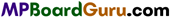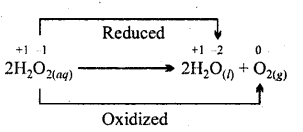These MP Board Class 11th Chemistry Notes for Chapter 8 Redox Reactions help students to get a brief overview of all the concepts.

## MP Board Class 11th Chemistry Notes Chapter 8 Redox Reactions

→ Redox reaction : When both oxidation and reduction reactions occur simultaneously.

→ Oxidising agent: Substance which accept electron and get reduced.

→ Reducing agent: Substance which donate electron and get oxidised.→ Electrode potential: Potential developed on electrodes by oxidation or reduction. Its value is different for different electrode.

→ Electromotive force (EMF): Potential difference of both electrodes in the cell.

→ Nernst equation : Equation which express relation of electrode potential with ionic concentration and temperature.
E = E°+ $$\frac{\mathrm{RT}}{n \mathrm{~F}}$$ln[Mn+(aq)]

→ Oxidation: Addition of oxygen/ electronegative element in a substance or removal of hydrogen/ electropositive element.

→ Reduction : Removal of oxygen/electronegative elements from a substance or addition of hydrogen/electropositive element.→ Reducing agent: Species which is oxidized.

→ Oxidising agent: Species which gets reduced is called oxidizing agent.

→ Redox reactions are classified into four groups :
1. Addition , 2. Decomposition, 3. Displacement, 4. Disproportionation reaction.

→ Disproportionation reaction: Such redox reaction in which a single species undergo oxidation and reduction. Example:In such reactions in active species a single element obtains at least three oxidation state.

→ Increase in oxidation state leads to oxidation and decrease in oxidation state leads to reduction.

→ Oxidation number of an element can never be more than its group number.

→ Electrochemical cell: It is such a combination in which oxidation and reduction indirectly as half equations and in the reaction, chemical energy gets converted to electrical energy.→ Electrolytic cell: It is such an arrangement in which electrical energy is supplied from an external source to produce chemical reaction.

→ Electrode potential (E) : Potential difference between metal electrode and metal ion in which the electrode is dipped is called electrode potential.

→ Standard Electrode Potential (E°): If Pressure is 1 atm and concentration of electrolyte is 1 M then electrode potential is known as standard electrode potential (E°). Lower the value of E°, stronger reducing is the electrolyte. Higher the E° value, stronger is the oxidizing nature. The table of orderly arrangement of elements on the basic of their E° values is called Electro chemical series.

→ Electromotive force (EMF) or cell potential: The potential difference between two half cells of a cell is called cell potential
cell = E°cathode – E°anode

→ If E°cell is positive, the reaction proceeds in forward direction. If Ecell is negative the reaction proceeds in backward direction.→ Redox couple : The pair formed in presence of oxidized and reduced forms of substances participating in oxidation and reduction half reactions is called redox pair.